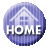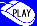Online Puzzle Divide by Box◆　Rules

Divide by Box is played on a rectangular grid. Some of the squares in the grid are numbered. The objective is to divide the grid into rectangular and square pieces such that each piece contains exactly one number, and that number represents the area of the rectangle.

◆　10 problems for beginners（Size of a cell 6x6 10problems）

50problems（Size of a cell 10x10 20problems,18x10 15problems,24x14 9problems,36x20 6problems）